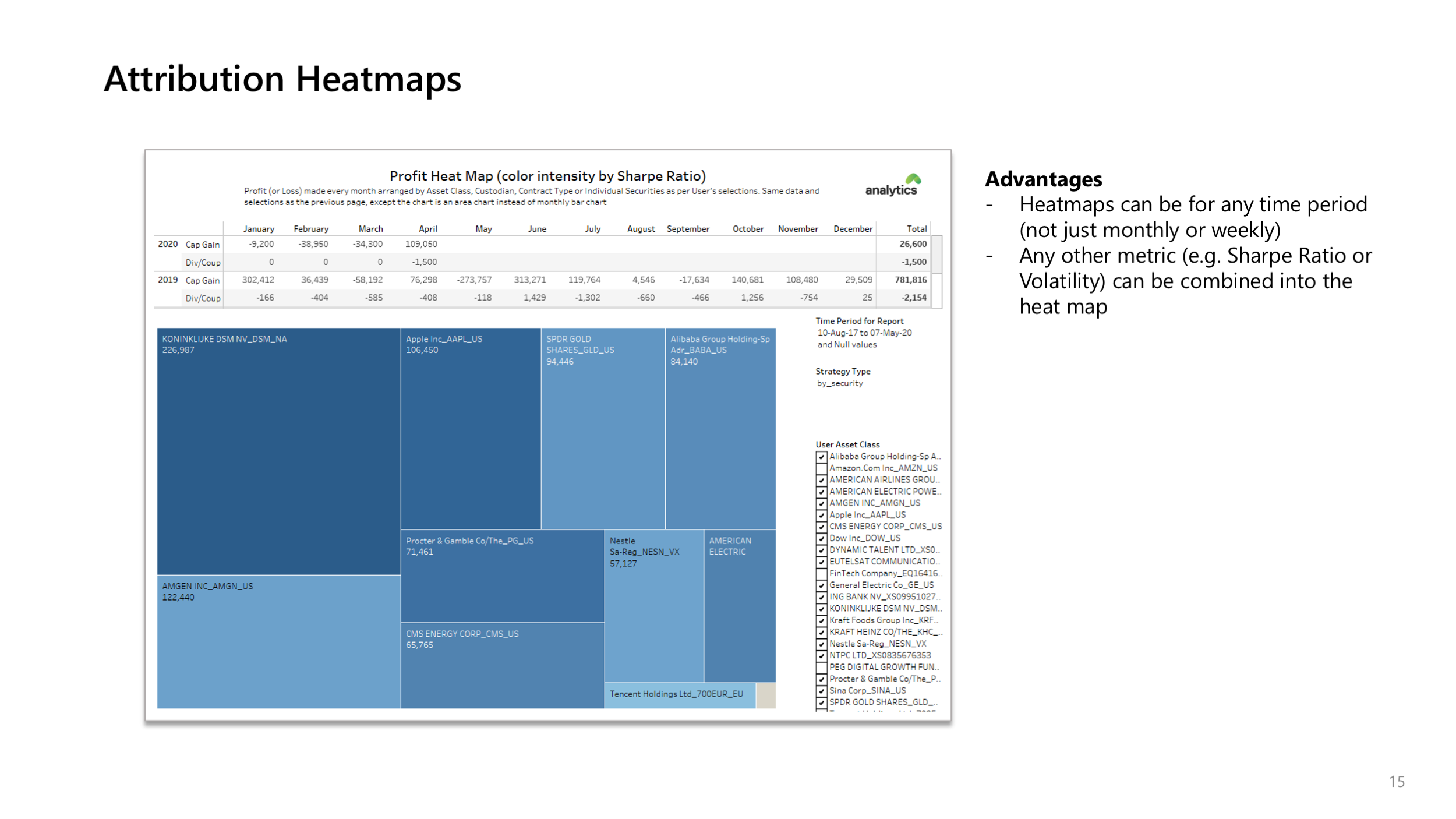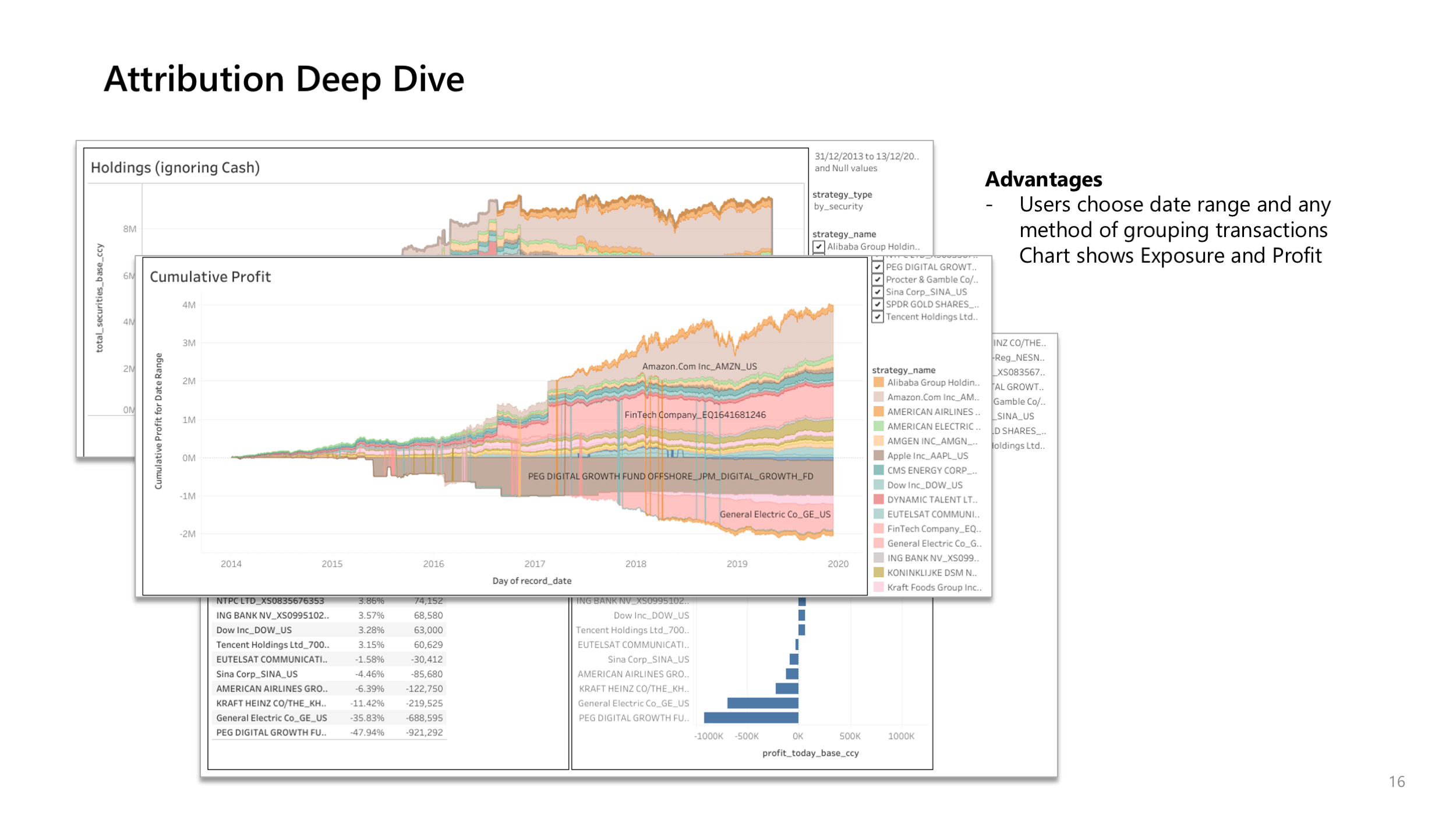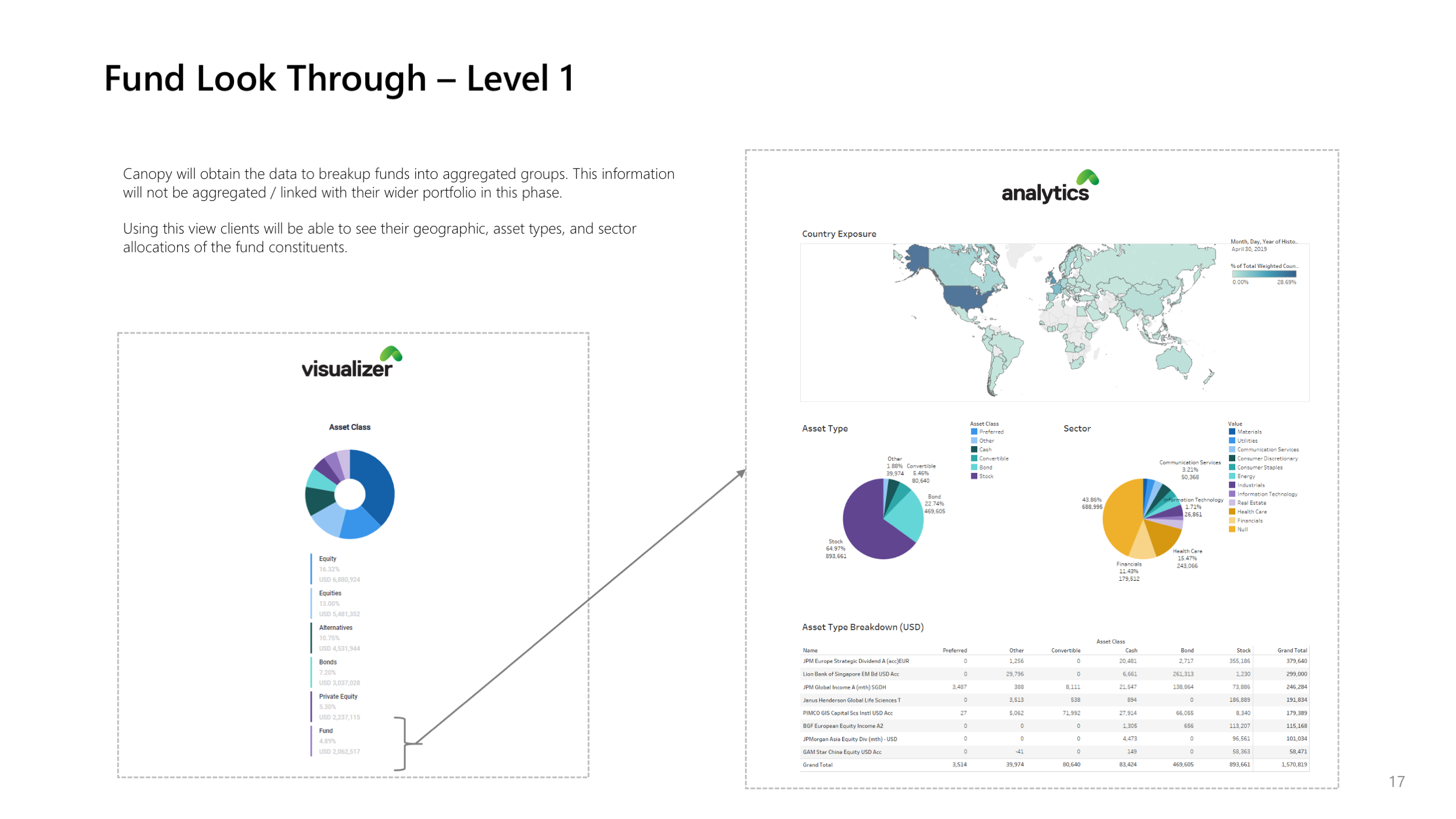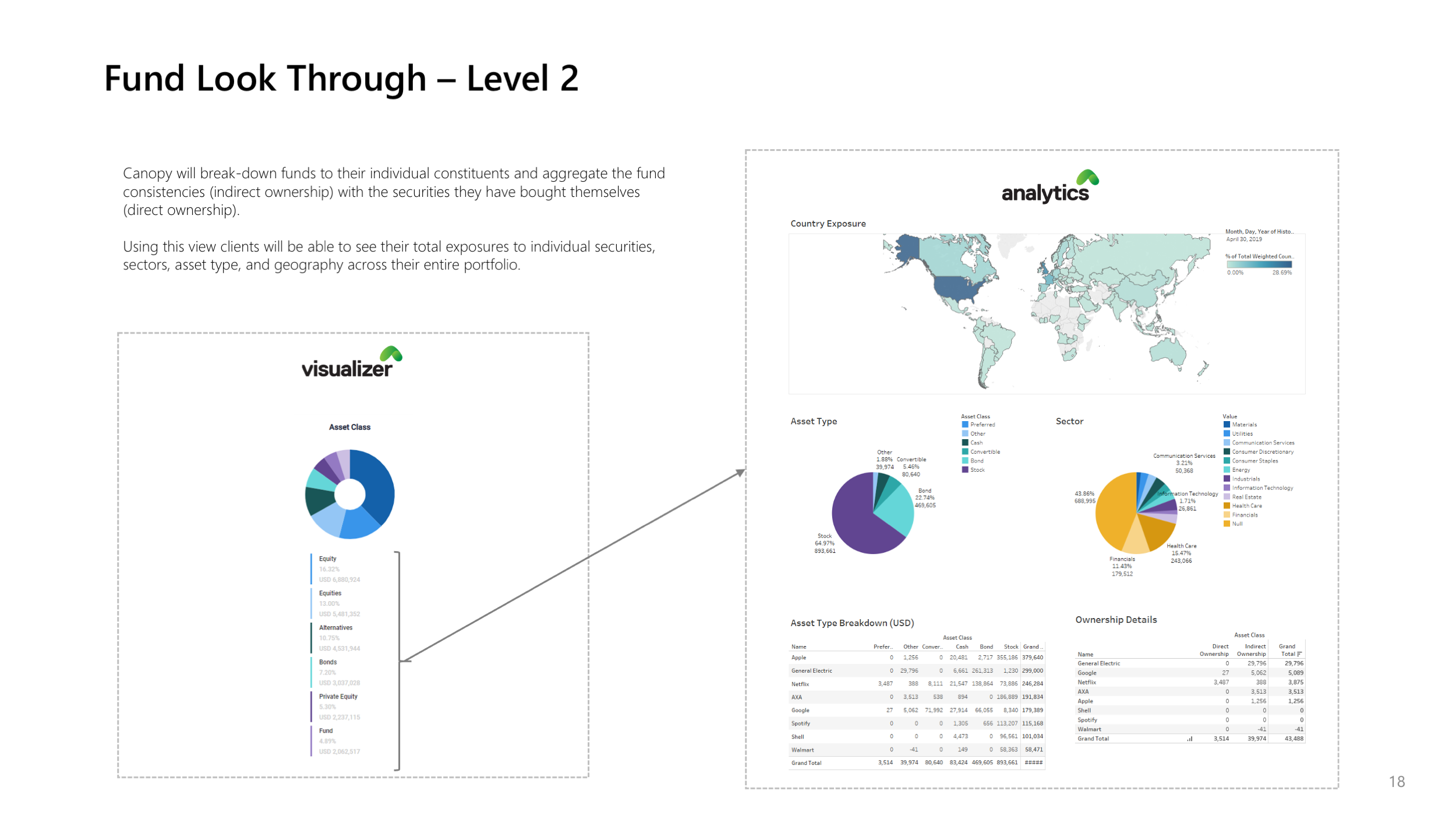# Financial Metrics Dashboards

## Asset Allocation

### Fully configurable Asset Classification

Users can choose to classify asset classes, sub-asset classes, geography as they wish

## Performance and Total Return

### Time Weighted Returns (TWR)

The time-weighted return (TWR) multiplies the returns for each sub-period or holding-period, which links them together showing how the returns are compounded over time.
The time-weighted return (TWR) helps eliminate the distorting effects on growth rates created by inflows and outflows of money.

Time Weighted Returns are calculated in the following methods

• TWR on Networth (i.e. Leveraged Returns)
• TWR on Non Cash Assets (i.e. ignores the funding cost of the strategy)
• TWR on Cash Deployed
• Unleveraged TWR

### Internal Rate of Return (IRR)

IRR, the performance metric of choice in the PE industry, represents the discount rate that renders the net present value (NPV) of a series of investments zero. IRR reflects the performance of a private equity fund by taking into account the size and timing of its cash flows (capital calls and distributions) and its net asset value at the time of the calculation.

### Total Return (including Coupons/Dividends)

Calculated daily, can be reported on any time frequency like daily, monthly, quarterly etc

### Realized and Unrealized Gains/Loss

Canopy uses Average Cost method to calculate Realized and Unrealized Gain/Loss. Detailed calculations are available

## Risk

### Mark to Market Risk

Fx and Price mark to market risk in your account can be visualized in multiple ways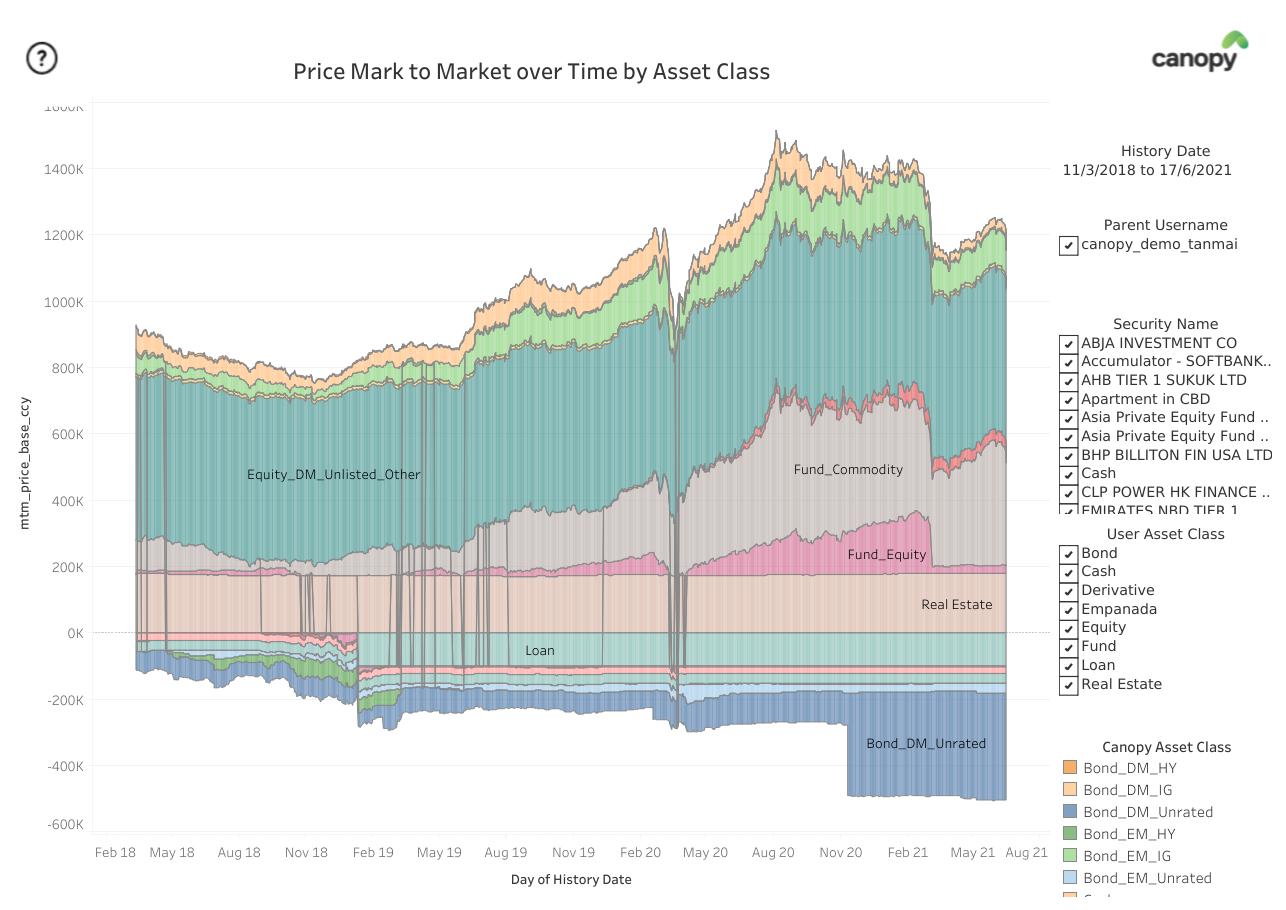Evolution of Price Mark to Market over time (similar chart for Fx Mark to Market or combined effect is possible)

### Value at Risk

Actual daily p&l volatility of the particular strategy is calculated. VaR is then estimated using a 95% confidence level and a normal distribution assumption

### Volatilty, Sharpe/Sortino Ratio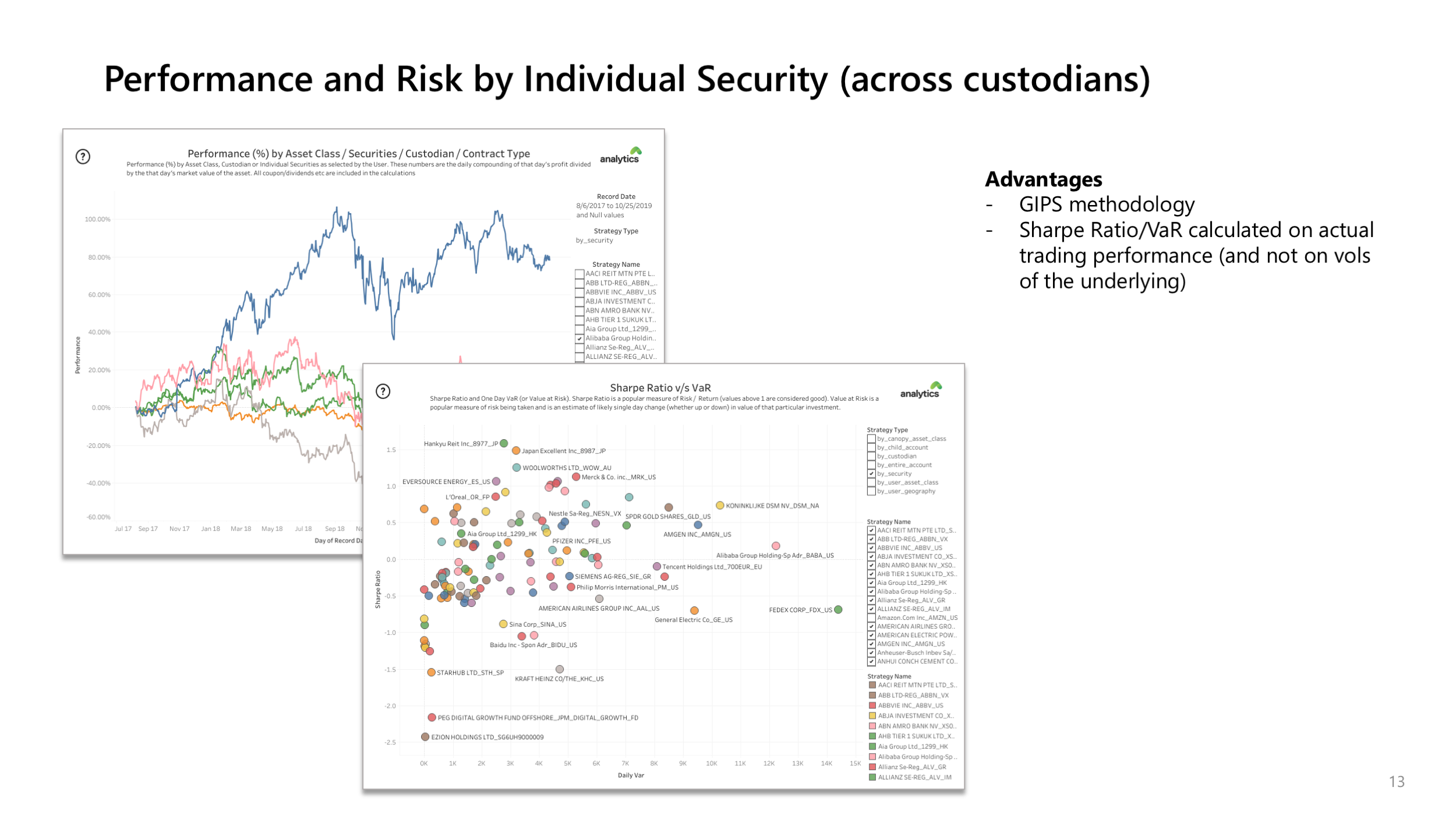Showing amount of profit and Sharpe Ratio (i.e. how much money was made and how well you made it) in one chart